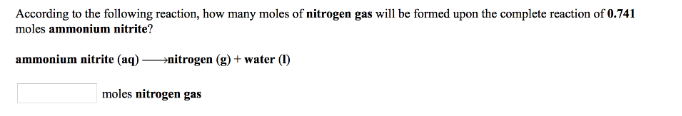# Problem: According to the following reaction, how many moles of nitrogen gas will be formed upon the complete reaction of 0.741 moles ammonium nitrite? ammonium nitrite (aq) → nitrogen (g) + water (l)

🤓 Based on our data, we think this question is relevant for Professor Kincaid's class at RICE.

###### FREE Expert Solution###### Problem Details

According to the following reaction, how many moles of nitrogen gas will be formed upon the complete reaction of 0.741 moles ammonium nitrite?

ammonium nitrite (aq) → nitrogen (g) + water (l)